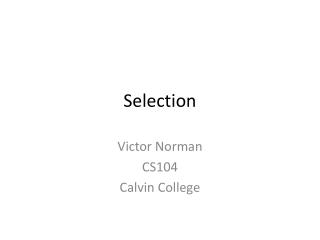DownloadDownload PresentationSelection

# Selection

Télécharger la présentation## Selection

- - - - - - - - - - - - - - - - - - - - - - - - - - - E N D - - - - - - - - - - - - - - - - - - - - - - - - - - -
##### Presentation Transcript

1. Selection Victor Norman CS104 Calvin College

3. New type: bool • Stands for Boolean (named after George Boole) • Two values: True and False • Operations: • and, or, not • equality (==), inequality (!=)

4. Booleans necessary for “testing” • You “constantly” are making decision: • if something is true, I’ll do this, otherwise, I’ll do that. • Code is similar. • if something < somethingelse: • something < somethingelse is a boolean expression: produces True or False. • if something == 0: • True if something evaluates to the value 0, False otherwise. • Can be used in assignments: • witch = (she == duck) # witch has value True or False.

5. Boolean operators • <val> < <val> • <val> > <val> • etc: <, >, <=, >=, ==, != Logical Operators: • <booleanValue> and <booleanValue> • <booleanValue> or <booleanValue> • not <booleanValue>

6. Clicker Questions

7. if Statement Syntax if <boolean expression>: <statements: executed if expr is True> if <boolean expression>: <statements: executed if expr is True> else: <statements: executed if expr is False>

8. Chained Conditionals if <boolean expression>: <statements: executed if expr is True> elif <boolean expression2>: <statements: executed if expr is False and expr2 is> elif <bool expr3>: <statements…> else: # optional! <statements>

9. Example of use Suppose you have a function called turnDegrees(deg) which takes a positive or negative value in deg. You want to turnLeft() if deg is positive, turnRight() if negative, and do nothing if 0. How would you write this code? if deg > 0: # this code in turnDegrees() turnLeft(deg) elif deg < 0: turnRight(-1 * deg)

10. Nested conditional Q: Write code that prints the word “odd” or “even” depending on the value of variable anInt, and also prints “greater than 10” if the odd number has a value greater than 10. A: if anInt % 2 == 0 : print "even” else: print "odd” if anInt > 10: print "greater than 10”

11. Clicker Questions

12. Assignment • Do ~60 questions in CodeLab. • Very good practice!